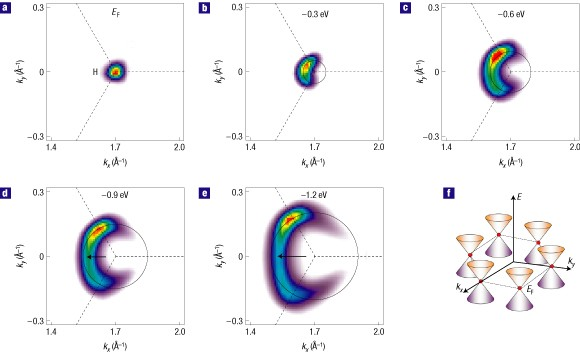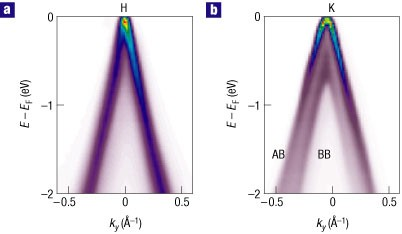## Main

For most condensed-matter systems, the physics is formulated in terms of the non-relativistic Schrödinger equation, and the low-energy excitations are quasiparticles with finite effective mass. For some special systems, for example, graphene/graphite, where the dispersion is expected to be linear near the Fermi energy, EF, and only touch EF at one point (Dirac point), the physics is described by the relativistic Dirac equation with the speed of light replaced by the Fermi velocity vF. The low-energy excitations in this case are Dirac fermions, which have zero effective mass and a vanishing density of states at the Dirac point. The Dirac fermions are proposed to be responsible for various anomalous phenomena observed in these systems1,2,3,8. So far, transport experiments in graphene have shown results in agreement with the presence of Dirac fermions1,2. Phase analysis of quantum oscillations in graphite has also suggested coexistence of both Dirac fermions and quasiparticles with finite effective mass9. Here, we report the first direct observation of massless Dirac fermions coexisting with quasiparticles with finite effective mass in graphite, by using angle-resolved photoemission spectroscopy (ARPES). ARPES provides the advantage of directly probing the electronic structure with both energy and momentum information, not accessible by any other measurement.

Figure 1a shows an ARPES intensity map measured near the Brillouin zone (BZ) corner H. The out-of-plane momentum, kz, is 0.5c*, where c* is the reciprocal lattice constant (see the Methods section for the extraction of kz values). Following the maximum intensity in this map, a linear Λ-shaped dispersion can be clearly observed. The dispersion can be better extracted by following the peak positions in the momentum distribution curves (MDCs), momentum scans at constant energies, shown in Fig. 1b. Here, two peaks in the MDCs disperse linearly and merge near EF. The Fermi velocity extracted from the dispersion is 0.91±0.15×106 m s−1, similar to a value 1.1×106 m s−1 reported by a magnetoresistance study of graphene2. We note that at low energy near EF, this linear dispersion is also observed along other cuts through the H point, with similar Fermi velocity. This linear and isotropic dispersion is in agreement with the behaviour of Dirac fermions.

Another way of probing the linear and isotropic dispersion is to study the intensity maps at constant energy. At EF (Fig. 2a), the intensity map shows a small object near H. The details of this small object will be discussed later. With increasing binding energy, this object expands and shows a circular shape (Fig. 2b,c). We note that only the circular shape in the first BZ is clearly observed. This is attributed to the dipole matrix element10, which suppresses or enhances the intensity in different BZs. However, taking the three-fold symmetry of the sample, this circular shape in the first BZ is expected to extend to other BZs and thus the electronic structure is isotropic near H. As the energy changes to −0.9 eV, the constant energy map deviates slightly from the circular shape (see the arrow in Fig. 2d). This deviation increases with binding energy and a trigonal distortion is clearly observed at −1.2 eV (Fig. 2e). This trigonal distortion is determined by the relevant tight-binding parameters for graphite and further studies to analyse this trigonal distortion are in progress. Overall, Fig. 2 shows that from EF to −0.6 eV, the electronic structure is isotropic in the kxky plane. Similarly, the Fermi velocity measured within the first BZ is 1.0×106 m s−1 with a ≤10% variation along different directions, consistent with the circular constant energy maps shown here. Combining the results of Figs 1 and 2, we conclude that from EF to −0.6 eV, the dispersion shows a cone-like behaviour near each BZ corner H, similar to that expected for graphene (Fig. 2f).Figure 2: Constant energy maps taken near the H point, showing that the electronic structure is isotropic in the kx–ky plane from EF to −0.6 eV.

To resolve the details of the low-energy dispersion and the small object at EF (Fig. 2a), we show in Fig. 3a an intensity map measured near H with lower photon energy to give better energy and momentum resolution. In the intensity map, two bands dispersing linearly towards EF can be distinguished, as is also clear in the MDCs (Fig. 3b) where two peaks can be observed for all binding energies. The extracted dispersion (open circles in Fig. 3a) from MDCs (Fig. 3b) shows two bands dispersing linearly towards EF, with a minimum separation of 0.020±0.004 Å−1 at EF. This linear dispersion near the H point, as well as the isotropic electronic structure shown in Fig. 2 from EF to −0.6 eV, is a basic characteristic of Dirac quasiparticles, which points to the presence of Dirac quasiparticles in the low-energy excitations near the H point in graphite. Furthermore, from the extracted dispersions, the Dirac point is extrapolated to be 50±20 meV above EF, and thus the small object observed at EF is a hole pocket, in agreement with previous studies of the three-dimensional band structure of graphite9,11. Assuming an ellipsoidal shape for the hole pocket12, the hole concentration is estimated to be 3.1±1.3×1018 cm−3, from the 0.020 Å−1 separation of the peaks at EF. This hole concentration is in agreement with reported values12,13,14. We note that, given the current resolution of the ARPES technique, we are not able to resolve the two hole pockets at the H point reported by the other experimental probe15. In fact, according to ref. 15, the difference in energy between these two hole pockets at the H point is ≤1 meV, which is beyond the current resolution of the ARPES technique. The presence of holes with Dirac fermion dispersion is further supported by the angle-integrated intensity (Fig. 3c), which is proportional to the two-dimensional density of states, barring the matrix element. In this energy range, a linear behaviour, similar to that expected for Dirac fermions, is observed. In addition, the energy intersect is at ≈50 meV above EF, in agreement with the Dirac point energy extrapolated from the dispersions.Figure 3: Detailed low-energy dispersion near the H point shows that low-energy excitations are Dirac fermions with the Dirac point slightly above EF.

Figures 13 show that near the H point, the low-energy excitations in graphite are Dirac fermions characterized by linear and isotropic cone-like dispersion, in agreement with transport measurements in graphite where Dirac fermions are suggested to coexist with quasiparticles that have finite effective mass9. To gain direct insight into the different types of quasiparticles, ARPES can provide a unique advantage by directly measuring the effective mass as well as accessing its momentum dependence. Figure 4a shows the intensity map near another high-symmetry point in the BZ corner, the K point. The dispersion (open circles) shows a parabolic behaviour, in sharp contrast to the linear behaviour observed near the H point (Figs 1, 3). This parabolic dispersion points to the presence of quasiparticles with finite effective mass. To determine the effective mass, we first extract the low-energy dispersion, then fit the MDC and energy distribution curve (EDC) dispersions (Fig. 3b,c) with a parabolic function. In both cases, the effective mass is determined to be 0.069±0.015me, where me is the free-electron mass. This effective mass measured by ARPES is different from the values reported by transport measurements13,14,16, 0.052 and 0.038me for electrons and holes respectively. This difference may be due to the fact that transport measurements are not momentum selective, and therefore the mass measured is the average mass over all kz values. On the other hand, ARPES is momentum selective and the value for the effective mass is only for this specific kz value. Taking this into account, the agreement between these measurements is reasonable. In summary, the data presented so far show that the low-energy excitations in graphite change from massless Dirac fermions with linear dispersion near H (Figs 13) to quasiparticles with parabolic dispersion and finite effective mass near K (Fig. 4a).Figure 4: Detailed dispersion near K, which shows that quasiparticles with finite effective mass and defect-induced localized states also contribute to the low-energy electronic dynamics.

We now discuss another interesting feature observed in graphite, that is, a large electron pocket near EF. Figure 4e shows the intensity map measured in the same experimental conditions as Fig. 4d except at a different spot. In Fig. 4e, a strong and large electron pocket within 50 meV below EF is the dominant feature. Weak signatures of the parabolic π band (as in Fig. 4d) can still be observed, as the MDC at EF (Fig. 4f) demonstrates. Here, in addition to the two main peaks (black arrows) corresponding to the electron pocket, a central weak peak (grey arrow) corresponding to the top of the parabolic band can also be distinguished. From the separation (≈0.1 Å−1) between the two main peaks at EF, the electron concentration is determined to be 8.0±0.7×1019 cm−3, which is an order of magnitude higher than the values reported13,14. Moreover, from the dispersion (Fig. 4e), the effective mass is extracted to be 0.42±0.07me, which is also much larger than any mass reported by transport measurements13,14,16.

This large electron pocket is observed in most of the samples measured, and thus it represents an important feature associated with graphite. We note that a similar large electron pocket has been reported recently and proposed to be associated with either edge states or dangling bonds12. Here we provide detailed characterization of this large electron pocket, which is important in revealing its origin. We propose defect-induced localized states as a possible explanation for this large electron pocket, on the basis of the following reasons. First, the electron concentration and effective mass measured for this electron pocket are much larger than reported values. Second, although the parabolic π band in Fig. 4a,d is observed in all the samples measured and in different spots (averaged over ≈100 μm) within the same sample, this large electron pocket strongly depends on the spot position within the same sample. Third, scanning tunnelling microscopy shows that zigzag edges can induce a peak in the local density of states at an energy (≈−0.03 eV) similar to the electron pocket observed here17. In fact, it has been shown that a low concentration of defects (for example, edge states, vacancies and so on) can induce self-doping to the sample18,19. If this interpretation is correct, then further studies on this large electron pocket may provide insights into the magnetic properties of nano-graphite ribbons, because it has been proposed that some defect-induced localized states are magnetic20,21.

## Methods

High-resolution ARPES data have been taken at Beamlines 12.0.1 and 7.0.1 of the Advanced Light Source in Lawrence Berkeley National Laboratory with photon energies from 20 to 155 eV with energy resolution from 15 to 65 meV. Data were taken from single-crystal graphite (Kish graphite for Fig. 3a,b and natural graphite for other figures) at a temperature of 25 K. Throughout this paper, the kz values are estimated using the standard free-electron approximation of the ARPES final state22,23,24. The inner potential needed for extracting the kz value was determined from the periodicity of the detailed dispersion at the Brillouin zone centre ΓA using a wide range of photon energies from 34 to 155 eV. The consistency of the kz values is confirmed by the degeneracy of the π bands near H and a maximum splitting near K (Fig. 5), both in agreement with band structure11,25,26. Note that although this large energy range is good for discussing the splitting of the π bands, it cannot capture the deviation from linear behaviour at the K point expected at low energy (as discussed in Fig. 4).Figure 5: Dispersions measured near H and K, showing the general consistency of the extracted kz values.